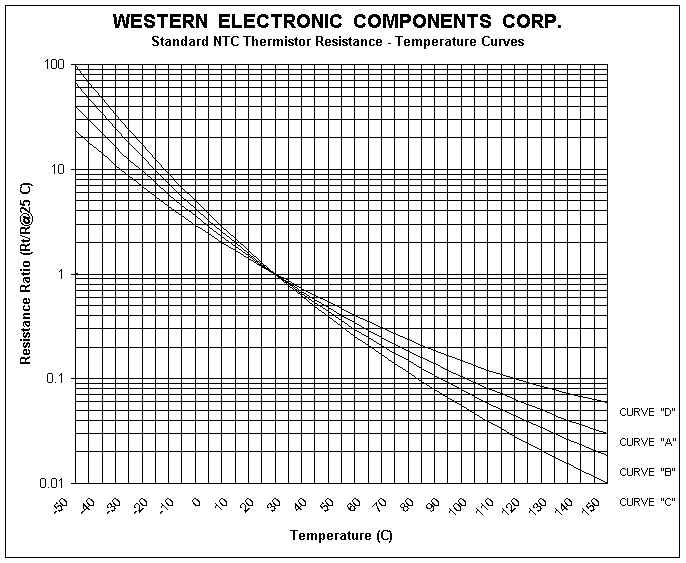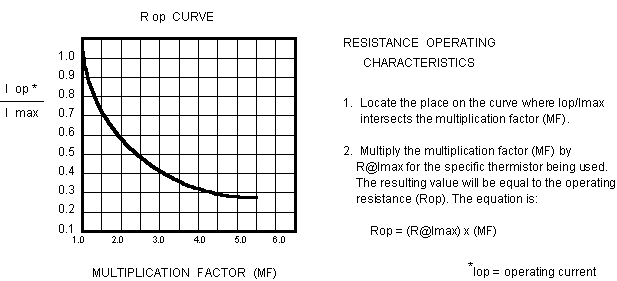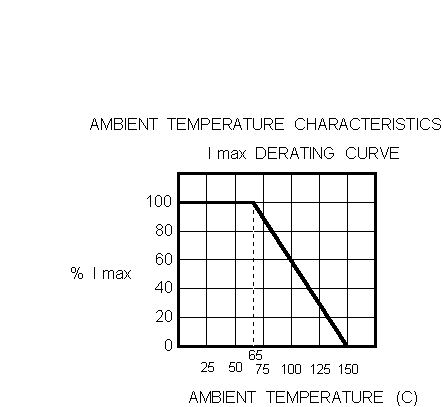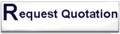NTC Engineering Note

TEMPERATURE MEASUREMENT

 The high sensitivity of a thermistor makes it an ideal candidate for low cost temperature measurement. One of the simplest applications is placing the thermistor in one of the legs of a wheatstone bridge. Substituting a second thermistor for Rx (fixed resistor) makes the circuit twice as sensitive, permitting the use of a lower sensitivity meter. Because the resistance vs. Temperature characteristic to the NTC thermistor is nonlinear, it is often advantageous to lineraize the curve. A simple voltage divider tends to linearize the output voltage as a function of temperature, while a single parallel resistor linearizes the resistance vs. Temperature curve. In each case, the maximum linearity error is a function of the length of the temperature span. For spans of less than 50 C, a single resistor can linearize to better than +/-0.5 C accuracy. Decreasing the total linearity error can be accomplished by using more than one thermistor in the network. If the temperature span is relatively short, it is also possible to improve accuracy by using a single thermistor in a series/parallel resistor network. THERMAL TIME CONSTANT The thermal time constant is an indication of the time that component needs to reach thermal equilbrium. This constant depends on two important parameters. One is the thermal capacity (H) of the component, the energy that must be applied to the component in order to raise its temperature by 1 Kelvin (or the energy that the component must lose in order to lower its temperature by 1 Kelvin). The units are thus quoted in Joules / Kelvin. The second parameter is called the dissipation factor. If the temperature of a component rises, it will tend to dissipate energy. This dissipation will depend on the surroundings and also on the component itself. The dissipation factor is defined as the ratio of the change in power dissipation with respect to the resultant body temperature change (units in W/K). TEMPERATURE  MEASUREMENT AND CONTROL When used in conjunction with an amplifier, the thermistor provides a low cost means of achieving highly reliable temperature control. The system can be simple as the on/off control of a transistor driving a relay or as sophisticated as a closed loop proportional controller. The thermistor's main asset in temperature control applications is its high degree of sensitivity. At 25 C a typical NTC changes about -4.4% in resistance for a 1C change in temperature. Using thermistors, temperatures have been controlled to better than 0.001 C.NTC INRUSH CURRENT LIMITERS (ICL) Inrush current limiters are used to solve the problem of current surges in switch mode power supplies. The current surges are created by large filter capacitors used to smooth the ripple in the rectified 60Hz (50Hz) current prior to being chopped at a high frequency. The relatively high initial resistance of the thermistor acts to limit the inrush current until the power it is dissipating heats it to a high temperature. At this high temperature, the very low resistance of the thermistor effectively removes it from the circuit. A typical switch mode power circuit is illustrated below. NTC INRUSH CURRENT LIMITERS (ICL) Inrush current limiters are used to solve the problem of current surges in switch mode power supplies. The current surges are created by large filter capacitors used to smooth the ripple in the rectified 60Hz (50Hz) current prior to being chopped at a high frequency. The relatively high initial resistance of the thermistor acts to limit the inrush current until the power it is dissipating heats it to a high temperature. At this high temperature, the very low resistance of the thermistor effectively removes it from the circuit. A typical switch mode power circuit is illustrated below.MAXIMUM SURGE CURRENT

The main purpose of limiting inrush current is to prevent components in series with the input to the switcher from being damaged. Typically, inrush protection prevents nuisance blowing of fuses or breakers as well as welding of switch contacts. Since most thermistor materials are very nearly ohmic at any given temperature, the minimum no load resistance of the thermistor is calculated by dividing the peak input voltage by the maximum permissible surge current in the power supply.

The maximum steady state current rating of a thermistor is mainly determined by the acceptable life of the final products for which the thermistor becomes a component. In the steady state condition, the energy balance reduces to a heat balance formula. As more current flows through the device, its steady state operating temperature will increase. So rather than being a problem of maximum current, the problem becomes one of maximum temperature. Western Electronic components Corp. (WECC) Inrush current limiters are designed to withstand high temperatures (> 180 C). This allows them to operate at high steady state current values.

The following R op Curve is used to determine the resistance value of the thermistor when operating at less than the maximum steady state current.The following I max derating curve is used to calculate the lower I max value when the ambient temperature rises.